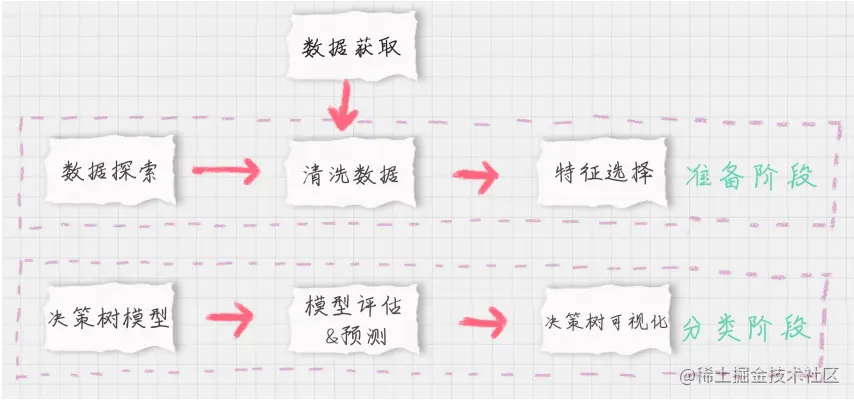# Titanic passenger survival prediction

2022-01-27 05:33:56

### Decision tree algorithm -

DecisionTreeClassifier(criterion='entropy')
criterion - standard entropy： Based on information entropy - namely ID3 Algorithm , The actual results are consistent with C4.5 Little difference
gini: The gini coefficient - namely CART Algorithm

### The key process of survival prediction1. Preparation stage

1. Data exploration - Analysis data quality
1. info - Basic information of the data sheet ： Row number 、 Number of columns 、 The data type of each column 、 Data integrity
2. describe - Statistics of the data sheet ： total 、 The average 、 Standard deviation 、 minimum value 、 Maximum
3. describe(include = [ 'O' ] String type ( The digital ) The overall situation of
4. head - The first few lines of data
5. tail - The last few lines of data
2. Data cleaning
1. Fill in missing values - mean value / Maximum frequency value
df.fillna(df['XX'].mean(), inplace = True)
df.fillna(df['XX'].value_counts(), inplace = True)
3. feature selection - Data dimension reduction , Facilitate subsequent classification operations
1. Filter out meaningless Columns
2. Filter out columns with more missing values
3. Put the remaining features into the feature vector
4. Data conversion - Convert character column to numeric column , Convenient follow-up operation - DictVectorizer Class to convert
DictVectorizer: Symbols are converted into numbers 0/1 To said
1. Instantiate a converter
devc = DictVectorizer(sparse = False) - sparse=False It means not using sparse matrices , A sparse matrix is a matrix in which a non - 0 Values are expressed by position
one-hot To make the category more equitable , There is no priority between each other
2. call fit_transform() Method
to_dict(orient='record') - convert to list form
2. Classification stage

1. Decision tree model
1. Import decision tree model
1. Generate decision tree
2. Fitting generates a decision tree
2. Model to evaluate & forecast
1. forecast - The decision tree outputs the prediction results
2. assessment Known predicted values and real results - clf.score( features , Results tab ) Don't know the real prediction results - K Crossover verification - cross_val_score
3. Decision tree visualization
3. Drawing stage - GraphViz

1. Install first graphviz
2. Import graphviz package - import graphviz
3. sklearn Import export_graphviz
4. First use export_graphviz The data to be displayed in the decision tree model
5. Reuse graphviz Acquisition data source
6. Data presentation

K Crossover verification
Take out most of the samples for training , A small amount is used for classifier verification - do K Secondary cross validation , Every time you select K One third of the data is verified , The rest is for training , take turns K Time , Average.

• Divide the data set evenly into K Equal parts

• Use 1 Data as test data , The rest is training data

• Calculate the test accuracy

• Use different test sets , repeat 2、3 step